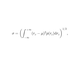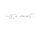# Correlation with P-Value & Confidence Interval [pig]

4743 views
Pearson correlation coefficient measures the linear correlation between two variables. It has a value between +1 and −1, where 1 is total positive linear correlation, 0 is no linear correlation and −1 is total negative linear correlation. It’s often denoted by r for sample correlation and ρ for population correlation.

Note: Pearson Correlation only measures the linear relationship between two variables, such as y = a*x + b. There are other measurements for nonlinear correlations.

When option “R” for "Correlation variants" is chosen, the value would be the same as TradingView's built in correlation() function. For "Adjusted R ", the calculation is based on the traditional Pearson. The sample r is a biased estimate of population ρ. The adjusted r gets rid of some of the bias but not all. As the sample size or lookback period increases, adjusted r will be closer to r.

The confidence interval is computed for population ρ estimation based on sample r. Correlation coefficient itself doesn’t follow a normal distribution. Fisher transformation is applied to transform the data into an approximately normal distribution. We compute the standard error based on the transformed data, then use an inverse fisher transform to transform back the standard error in terms of r.

Note: the confidence interval band is an approximation of population, it proposes a range of plausible r values (instead of a point). The confidence level represents the frequency (i.e. the proportion) of possible confidence intervals that contain the true value of the unknown population parameter. The proportion of those intervals that contain the true value of the parameter will be equal to the confidence level. For example, if the confidence level is 95% then in hypothetical indefinite data collection, in 95% of the samples the interval estimate will contain the population parameter. The default setting is 1.96* standard error which is 95% confidence interval.

The most important and distinguishable feature of this indicator is the p-value provided along with the correlation.

The value of Correlation Coefficient alone doesn’t provide any information regarding its statistical significance. For example, two sets of independent samples have 0 correlation in theory. However, your correlation coefficient on these samples will never actually show 0 correlation (small correlation value but not 0). Therefore without a significance test, one would be fooled by the value of r when there’s no linear relationship at all.

In statistical hypothesis testing, the p-value or probability value is the probability of obtaining test results at least as extreme as the results actually observed during the test, assuming that the null hypothesis is correct. The smaller the p-value, the stronger the evidence that the null hypothesis should be rejected and that the alternate hypothesis might be more credible. Since one could be deceived by r showing values while correlation is actually 0. The null hypothesis here is the “r is 0”. The alternative hypothesis is “ r is not 0”. The default setting for p critical value is 0.05. It means that when p is lower than 0.05, there’s less than 5% chance that correlation is 0, and we consider that to be "significant correlation". To get the p-value, We use a t distribution with n – 2 degrees of freedom to find the probability. P-value will adjust automatically when the sample size or lookback changes.

Displays :
When p is lower than 0.05 and r > 0, correlation coefficient shows red, p-value shows yellow, panel shows “Significant Positive Correlation”.
When p is lower than 0.05 and r < 0, correlation coefficient shows green, p-value shows yellow, panel shows “Significant Negative Correlation”.
When p is higher than 0.05, correlation, correlation coefficient shows white, p-value shows grey, panel shows “Insignificant Correlation”.

r² (r squared) also known as the coefficient of determination, is the square of correlation r. r² measures how well the data fit the linear regression model used in correlation. When two assets show significant correlation, r squared can be used to compare which one fits the data better. r² is displayed on the panel and has a different lookback by default than the correlation coefficient .

Contributors : Pig (ideas, code, math and design), Balipour (ideas), midtownsk8rguy(applying/employing Pine etiquette).
Versionshinweise: minor fix to adjust panel's positioning.
Versionshinweise: added feature to assist the visually impaired.
Versionshinweise: Modified the security() function to avoid lookahead bias.
Versionshinweise: removed the mtf function.
Versionshinweise: update to modify default position of the panel.

## KommentareThis publication will be featured in our "Best Scripts of The Month" selection. Thank you for your valuable contribution to the TradingView community, and congrats!
AntwortenDefinetly mind bending eplanation for non math geniuses such as myself :) . Folks won't have to argue anymore about two assets being correlated or not so once again, Bali, you have made the world a better place. Thank you Balipour, the Pig and anyone else who contributed to making past, present and future scripts and sharing them!
Antwortencarpe_diem_canis
@carpe_diem_canis,thank you. as far as we know this is the only CC that provides p-value (plus CI and r²). a cc without p value is irrelevant noise and potentially harmful
AntwortenI'm sure this is an amazing indicator and I've heard great things about the developers and love pig's ISI EMA. BUT the technicals on this one is so far above my layman thinking that I have no idea how to use it.
@balipour, is there any sort of tutorial of video on YouTube that shows how to use this indicator?? Appreciate it.
Antworten